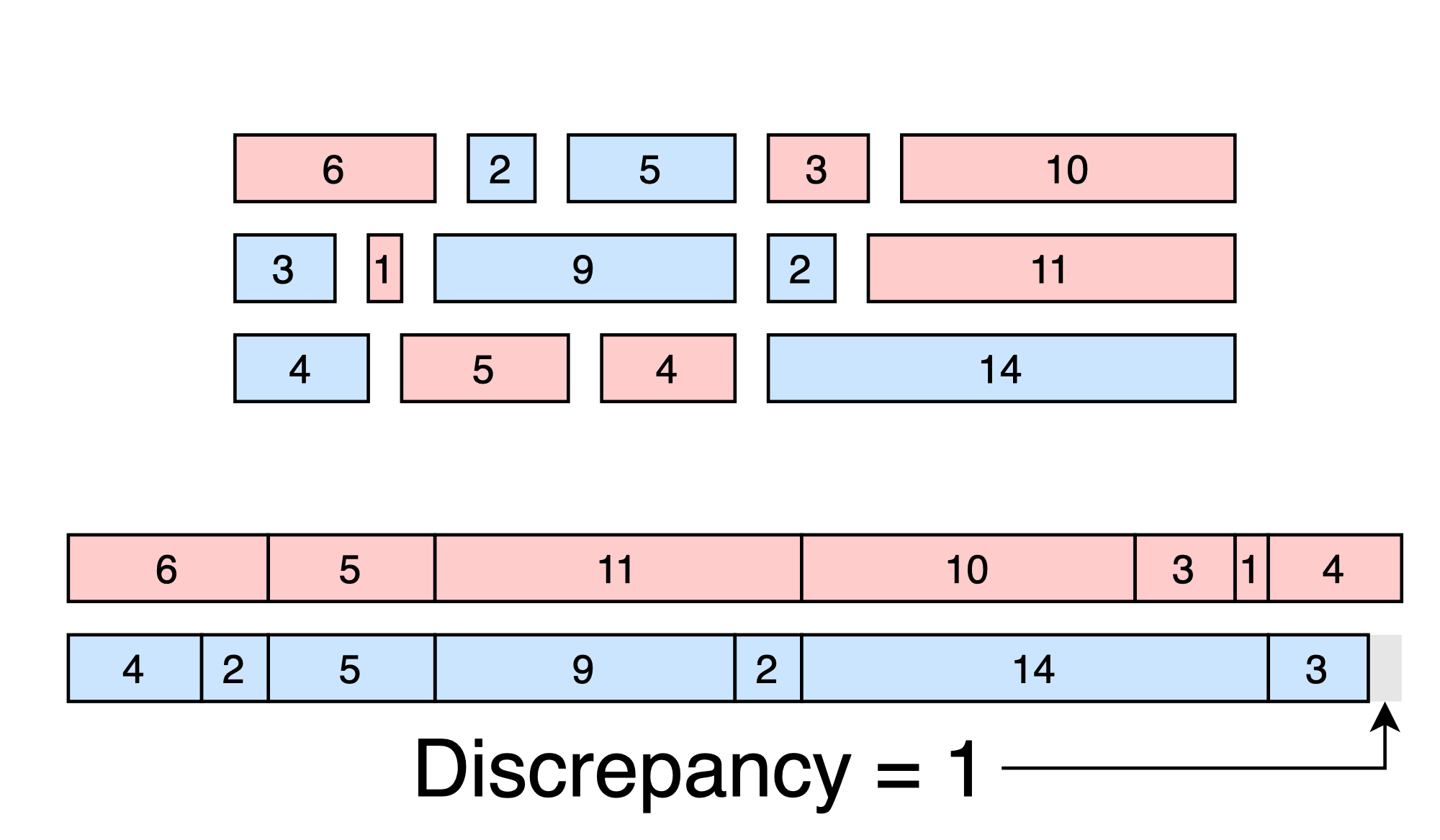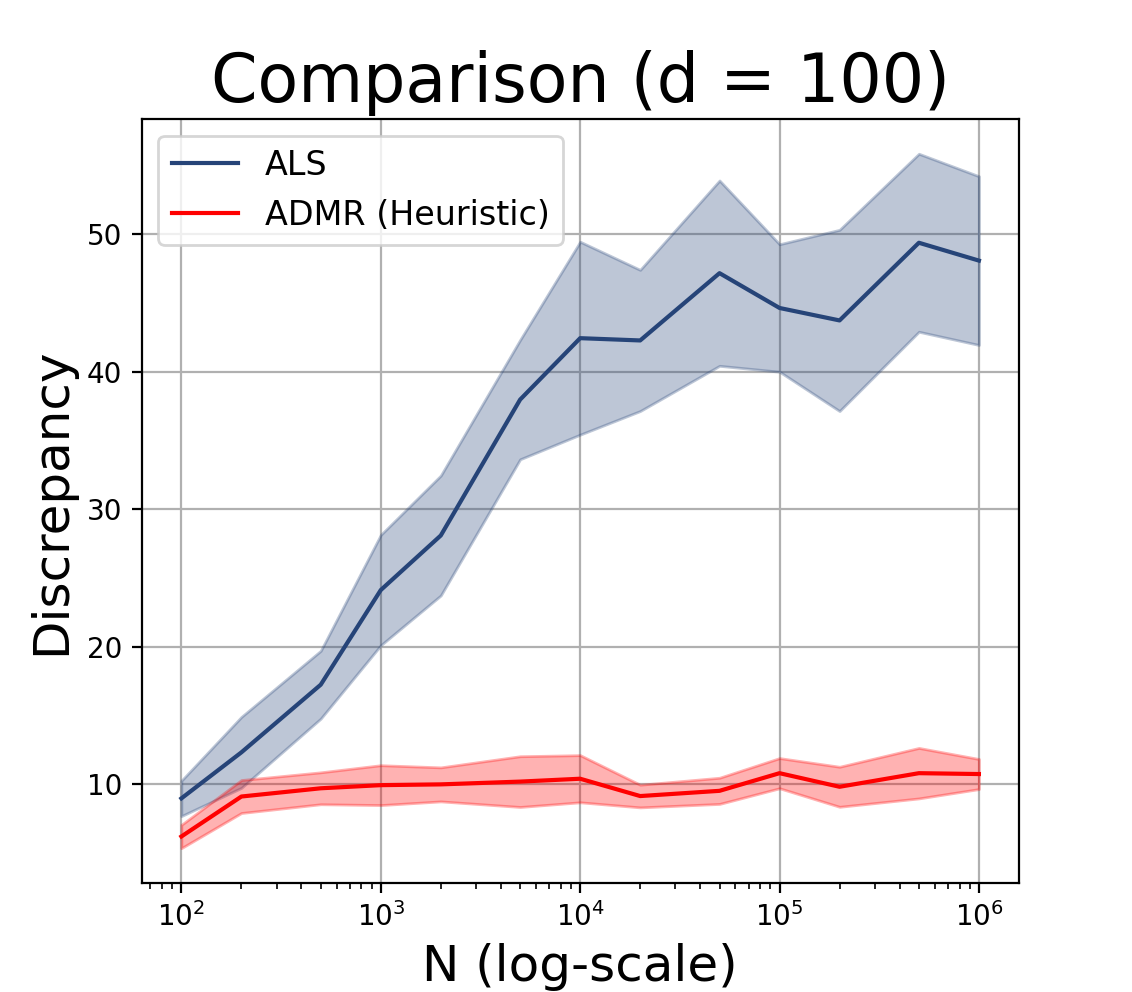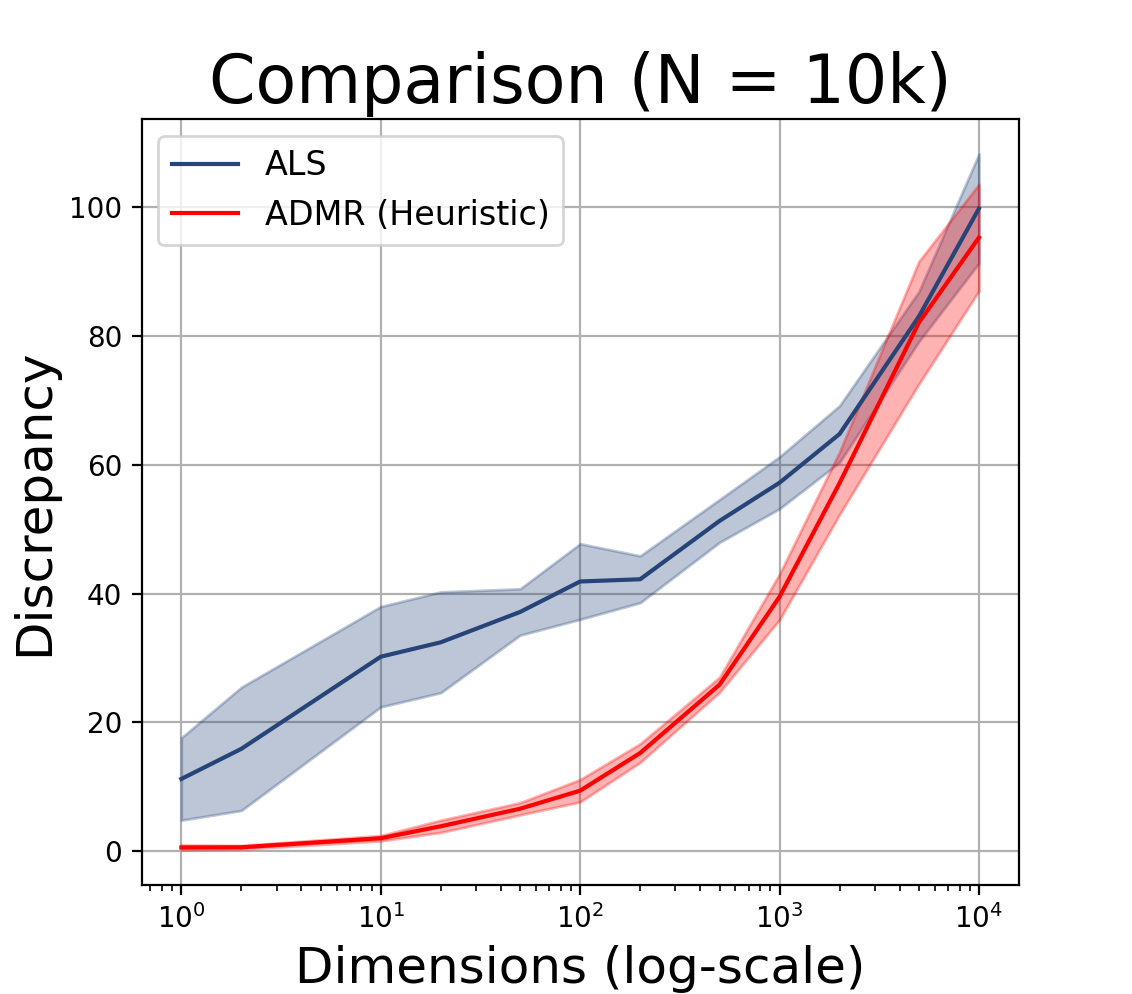# Streaming Algorithms for Discrepancy Minimization

Posted by Ben Coleman on September 05, 2021 · 13 mins read

Minimizing discrepancy is a core part of several recent proposals for efficient machine learning and dataset summarization. Unfortunately, the problem is NP hard, and we are forced to use approximate solutions.

Some of these approximations are expensive, but they don’t have to be. Thanks to new research on the problem, we can now do a reasonably good job in a single pass through the data.

This post is aimed at practitioners who want to try out machine learning algorithms involving discrepancy but don’t want to solve hard optimization problems to do it. I’ll talk about two recent methods that I’ve used with good success. The algorithms are fast even for million-scale datasets, are super simple and can be implemented in under 10 lines of Python.

## Discrepancy Minimization Problem

We’re going to consider the following discrepancy minimization problem:

Discrepancy Minimization: Given a set of d-dimensional vectors $$x_1, x_2, ... x_N$$, choose signs $$s_1, s_2, ... s_N$$ to minimize the discrepancy:

$\left\|\sum_{n = 1}^N s_n x_n \right\|_{\infty} = \max_d \sum_{n = 1}^N s_n x_{N}[d]$

In other words, we want to break the set of N points into two optimally balanced groups - one with sign +1 and the other with sign -1.If our points were all in one dimension, discrepancy minimization might look like this. We divide the N points into two groups that balance the numbers in each group. In higher dimensions, we have to juggle multiple parallel versions of this problem (one for each dimension). This is hard because we have to find a single partitioning that solves d simultaneous instances of the problem.

### Discrepancy for Machine Learning

This idea of discrepancy is different from the one that’s used in the construction of coresets, loss estimators, fast neural networks and other machine learning applications. In machine learning, we actually care about how the groups differ in terms of the loss for a model $$\theta$$.

$\left\|\sum_{n=1}^N s_n \mathrm{loss}(x_n,\theta)\right\|$

However, the two ideas are closely related. In practice, I’ve found that the mismatch doesn’t really matter - you get roughly the same results.

For models where the loss is a function of the inner product $$\langle x_n, \theta\rangle$$, you can make this relationship explicit by looking at the Taylor expansion of the loss function. If you’re interested, see Lemma 17 of this paper.

## Discrepancy Minimization via a Self-Balancing Walk

Alweiss, Liu and Sawhney published a paper in STOC 2021 that directly addresses the streaming discrepancy problem.1 Their randomized algorithm comes with strong theoretical guarantees and (to my knowledge) is the first to rigorously solve the problem in $$O(N)$$ time.

What’s more, their method is extremely easy to implement. This is a pleasant surprise, since algorithms presented at STOC and FOCS aren’t always easy to translate to practice.2 Here is an implementation in Python.

### Explanation of the Algorithm

The algorithm uses a “memory vector” $$w$$ that remembers the running totals for each dimension. We want each dimension of the memory vector to be as close to zero as possible. If the discrepancy starts to get too big for a particular dimension, the corresponding entry of $$w$$ will be large as well. We use this information to pick a sign that will push $$w$$ back towards zero - in other words, we use a self-balancing random walk.

## Better Practical Results with Heuristics

The previous algorithm works well and is efficient, but the large value of c causes it to frequently pick the same sign early in the algorithm. I learned from Tung Mai and Anup Rao3 that good heuristic is to simply set c = 1.

Unfortunately, this breaks the theoretical analysis in ways that seem difficult to fix. There is a paper describing the heuristic, but the theoretical issues remain open.

The algorithm is exactly the same as before, but now we do not have to know the number of items, N, in the data stream. We also don’t have to set the failure probability. Overall, this version is practically much more useful because the user does not have to set any parameters.

## Experimental Comparison

To see how well the algorithms do, I ran them on problems of varying size and dimension. As the input, I used N random points inside the d-dimensional unit hypercube. As the output, I measured the discrepancy found by the algorithm (lower is better). I report the mean and standard deviation over 10 experiments, where the randomness is over both the algorithm and the uniformly sampled dataset.The plot shows the result of running the discrepancy minimization methods on 100-dimensional input data. As we sweep N from 100 to 1,000,000, the gap between the ALS algorithm and the heuristic becomes larger.The plot shows the result of running the discrepancy minimization methods on a dataset of 10,000 points. As we sweep the dimensionality of the problem from 1 to 10,000, the problem becomes harder and the discrepancy increases.

## Theoretical Guarantees

This section is not terribly important from a practical perspective, but it’s interesting and possibly useful (if you’re using these algorithms as a building block for other applications). The theoretical objective is to prove that the signs chosen by the algorithm result in low discrepancy.

Given any set of N vectors, the lower bound for the problem is at least:

$\Omega \left(\sqrt{\frac{\log N }{\log \log N}}\right)$

This means that no matter what algorithm we pick, there will always be adversarial problem instances where we cannot get smaller than (roughly) $$\log N$$ discrepancy. The algorithm from STOC 2021 has discrepancy:

$O\left(\log \left(\frac{Nd}{\delta}\right) \right)$

where $$\delta$$ is the failure rate. The streaming algorithm is fairly close to optimal - only a factor of $$\sqrt{\log N}$$ away. The heuristic doesn’t have any guarantees, but it works better in practice. I suspect that for specialized problem instances, there are other heuristics that work even better. It would be interesting to see if provable statements can be made about the heuristic methods if we restrict our attention to specific problem instances, such as uniform or Gaussian distributions for x.

## Conclusion

Discrepancy minimization is a core subroutine in a number of recent algorithms in machine learning. For example, coresets often involve some kind of discrepancy-minimizing partition. We introduced two efficient ways to solve this problem that work on streaming data and don’t need lots of RAM. If you encounter a use case for discrepancy minimization, consider using one of the methods.

1. The paper is called, “Discrepancy Minimization via a Self-Balancing Walk” and is by Ryan Alweiss, Yang Liu and Methaab Sawhney.

2. This is not meant to criticize the theoretical computer science community - their goal isn’t to solve practical problems. It’s to advance our understanding of fundamental problems. And STOC/FOCS papers often contain “hidden gems” that can develop into a practical algorithms.

3. I had the good fortune to work with them at Adobe Research during my PhD. It was a pleasure to work there and I learned a lot.